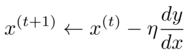Simple Linear Regression

20160120

## Minimize the Residual Sum of Squares

The slope & intercept can also be found via minimizing the RSS, which is a function of the slope (w₁) and intercept (w₀):Finding the minimum or maximum corresponds to setting the derived function equal to zero.

KLAD For a concave or convex function there is only one point where there is a maximum or minimum, ie where the derivative equals zero. If a function is not concave nor convex, then it may have multiple maxima or minima.

## Side-step: hill descent

The gradient descent algorithm tries to find a minimum, in a step-wise fashion. This can easily be compared to the following hill descent for a simple parabole function, eg. `y = 5 + (x-10)²`.

### Hill descent with overshoot

Suppose you start at point x=1. To see if the function is going down or up we add a minute quantity, dx, and know that we are descending if f(x) > fx(x+dx).

Increment x with step=15.0, and look again at the direction in which the function is going. Here the direction has flipped, we have overshot our target, so for the next step we need to divide the step-size into half. Etc.

Keep doing this until the difference is smaller than a predefined constant.To reach the result of 9.99999773021 required 57 iterations.

 ```12 13 14 15 16 17 18 19 20 21 22 23 24 25 26 27 28 29 30 31 32 33 34 35 36 ``` ``````step=15.0 # increment dx=1e-10 # miniscule value to check direction with, and to compare step with x0=1.0 # start x x0d=is_down(x0,x0+dx) # true if from x0 to x0+dx is going downhill sgn=1.0 # add or subtract pt_v=[] # store points in a list, for plotting pt_v.append( (x0,fx(x0)) ) count=0 while step>dx: x1=x0+sgn*step x1d=is_down(x1, x1+dx) if x0d!=x1d: # direction changed means overshoot step=step/2.0 # divide step by half sgn=1.0 if x1d else -1.0 x0=x1 x0d=x1d pt_v.append( (x0,fx(x0)) ) count+=1 print "Iterations: ", count," Result: ", x0``````

### Hill descent without overshooting

The following algo is similar, but avoids overshooting: in case we overshoot, it keeps adjusting the step-size until we land on the left side of the minimum.This time to get the result of 9.99999773022 required only 21 iterations, quite a bit better than above, but that of course may be due to the function and the choosen stepsize.

 ```12 13 14 15 16 17 18 19 20 21 22 23 24 25 26 27 28 29 30 31 32 33 34 ``` ``````step=15.0 # increment dx=1e-10 # miniscule value to check direction with, and to compare step with x0=1.0 # start x x0d=is_down(x0,x0+dx) # true if from x0 to x0+dx is going downhill pt_v=[] # store points in a list, for plotting pt_v.append( (x0,fx(x0)) ) count=0 while step>dx: # keep dividing step by two until there is no overshoot while True: x1=x0+step x1d=is_down(x1, x1+dx) if x0d==x1d: # same direction break step=step/2.0 x0=x1 x0d=x1d pt_v.append( (x0,fx(x0)) ) count+=1 print "Iterations: ", count," Result: ", x0``````

## The common functions for above code

### Function and direction

 ```5 6 7 8 9 10 ``` ``````# function (parabola) def fx(x): return 5 + (x - 10)**2 def is_down(x1, x2): return True if fx(x1)>fx(x2) else False``````

### Plotting

 ```5 6 7 8 9 10 ``` ``````# function (parabola) def fx(x): return 5 + (x - 10)**2 def is_down(x1, x2): return True if fx(x1)>fx(x2) else False``````

## Mathematical approach

The above 2 solutions are the naive implementations, that perform fairly well. The mathematical way would be to calculate the next x as:with step η typically chosen as 0.1.

During the ensuing iterations η can be kept constant or decreasing eg.Choosing the stepsize is an art!